Documentation

### This is machine translation

Mouseover text to see original. Click the button below to return to the English version of the page.

Note: This page has been translated by MathWorks. Click here to see
To view all translated materials including this page, select Country from the country navigator on the bottom of this page.

## Modal Analysis of a Flexible Flying Wing Aircraft

This example shows computation of bending modes of a flexible wing aircraft. The vibration response of the wing is collected at multiple points along its span. The data is used to identify a dynamic model of the system. The modal parameters are extracted from the identified model. The modal parameter data is combined with the sensor position information to visualize the various bending modes of the wing. This example requires Signal Processing Toolbox™.

### The Flexible Wing Aircraft

In this example, we study the data gathered from a small flexible flying wing aircraft built at the Uninhabited Aerial Vehicle Laboratories, University of Minnesota . The geometry of the aircraft is shown below.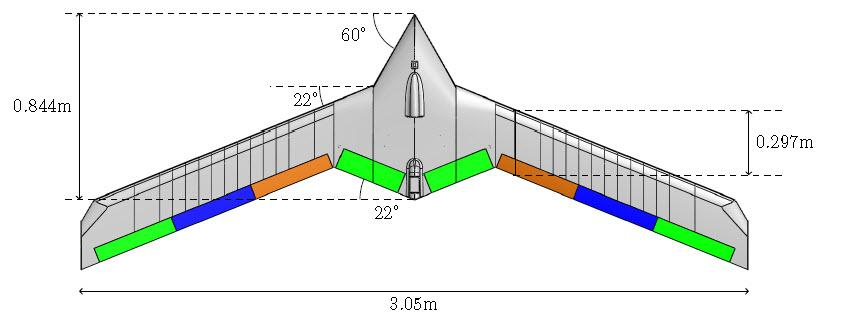The aircraft wing can undergo large deformations under loading. The flexible mode frequencies are lower than those in common aircraft with more rigid wings. This flexible design reduces material costs, increases agility and the flight range of the aircraft. However, if not controlled, the flexible modes can lead to catastrophic aeroelastic instabilities (flutter). Designing effective control laws for suppressing these instabilities requires accurate determination of the wing's various bending modes.

### Experimental Setup

The objective of the experiment is to gather vibration response of the aircraft at various locations in response to an external excitation. The aircraft is suspended from a wooden frame using a single spring at its center of gravity. The spring is sufficiently flexible so that the natural frequency of the spring-mass oscillation does not interfere with the fundamental frequencies of the aircraft. An input force is applied via an Unholtz-Dickie Model 20 electrodynamic shaker near the center of the aircraft.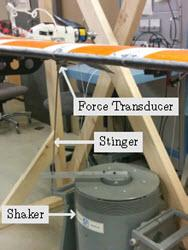Twenty PCB-353B16 accelerometers are placed along the wing span to collect the vibration response as shown in the next figure.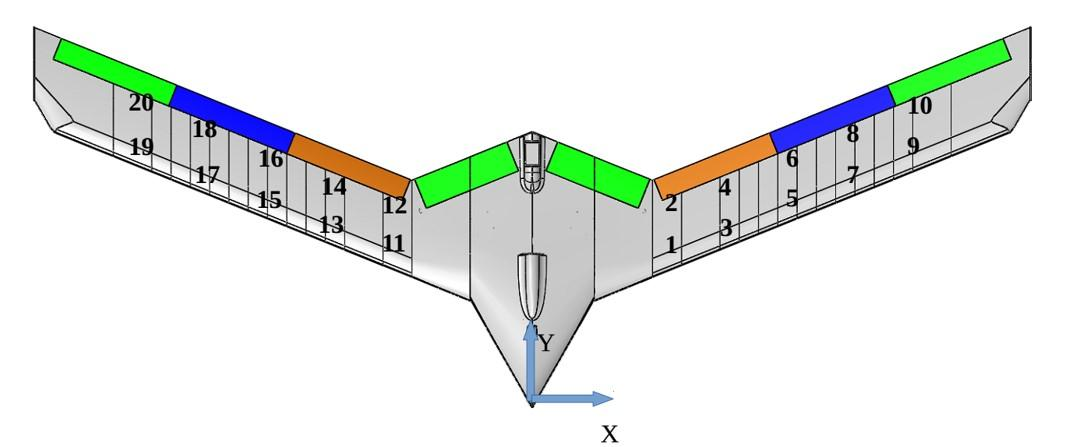The shaker input command is specified as a constant amplitude chirp input of the form $\mathit{A}\text{\hspace{0.17em}}\mathrm{sin}\left(\omega \left(\mathit{t}\right)\mathit{t}\right)$. The chirp frequency varies linearly with time, that is, $\omega \left(\mathit{t}\right)={\mathit{c}}_{0}+{\mathit{c}}_{1}\mathit{t}$. The frequency range covered by the input signal is 3–35 Hz. The data is collected by two accelerometers (leading and trailing edge accelerometers at one x-location) at a time. Hence 10 experiments are conducted to collect all the 20 accelerometer responses. The accelerometer and force transducer measurements are all sampled at 2000 Hz.

### Data Preparation

The data is represented by 10 sets of two-output/one-input signals, each containing 600K samples. Load the data from MAT file `FlexibleWingData.mat`:

`load FlexibleWingData.mat MeasuredData`

The variable `MeasuredData` is a structure with fields `Exp1`, `Exp2`, ..., `Exp10`. Each field is a structure with fields `y` and u containing the two responses and the corresponding input force data. Plot the data for the first experiment.

```fs = 2000; % data sampling frequency Ts = 1/fs; % sample time y = MeasuredData.Exp1.y; % output data (2 columns, one for each accelerometer) u = MeasuredData.Exp1.u; % input force data t = (0:length(u)-1)' * Ts; figure subplot(211) plot(t,y) ylabel('Outputs (m/s^2)') legend('Leading edge','Trailing edge') subplot(212) plot(t,u) ylabel('Input') xlabel('Time (seconds)')```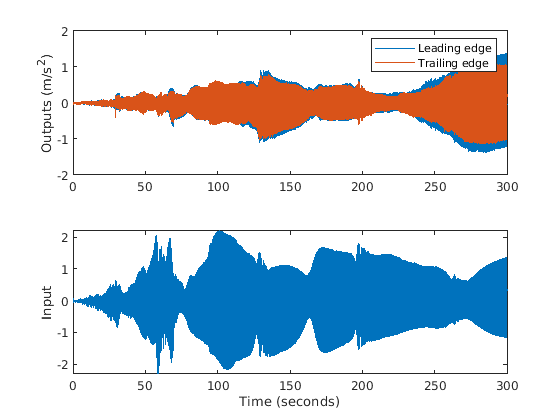In order to prepare data for model identification, the data is packaged into `iddata` objects. The `iddata` objects are standard way of packaging time-domain data in System Identification Toolbox™. The input signal is treated as bandlimited.

```ExpNames = fieldnames(MeasuredData); Data = cell(1, 10); for k = 1:10 y = MeasuredData.(ExpNames{k}).y; u = MeasuredData.(ExpNames{k}).u; Data{k} = iddata(y, u, Ts, 'InterSample', 'bl'); end```

Merge the dataset objects into one multi-experiment data object.

`Data = merge(Data{:})`
```Data = Time domain data set containing 10 experiments. Experiment Samples Sample Time Exp1 600001 0.0005 Exp2 600001 0.0005 Exp3 600001 0.0005 Exp4 600001 0.0005 Exp5 600001 0.0005 Exp6 600001 0.0005 Exp7 600001 0.0005 Exp8 600001 0.0005 Exp9 600001 0.0005 Exp10 600001 0.0005 Outputs Unit (if specified) y1 y2 Inputs Unit (if specified) u1 ```

### Model Identification

We want to identify a dynamic model whose frequency response matches that of the actual aircraft as closely as possible. A dynamic model encapsulates a mathematical relationship between the inputs and outputs of the system as a differential or difference equation. Examples of dynamic models are transfer functions and state-space models. In System Identification Toolbox, dynamic models are encapsulated by objects such as `idtf` (for transfer functions), `idpoly` (for AR, ARMA models) and `idss` (for state-space models). Dynamic models can be created by running estimation commands such as `tfest` and `ssest` commands on measured data in either time-domain or frequency-domain.

For this example, we first convert the measured time-domain data into frequency response data by empirical transfer function estimation using the `etfe` command. The estimated FRF is then used to identify a state-space model of the aircraft's vibration response. It is possible to directly use time-domain data for dynamic model identification. However, FRF form of data allows compression of large datasets into fewer samples as well as more easily adjust estimation behavior to relevant frequency ranges. FRFs are encapsulated by `idfrd` objects.

Estimate a two-output/one-input frequency response function (FRF) for each data experiment. Use no windowing. Use 24,000 frequency points for response computation.

```G = cell(1, 10); N = 24000; for k = 1:10 % Convert time-domain data into a FRF using ETFE command Data_k = getexp(Data, k); G{k} = etfe(Data_k, [], N); % G{k} is an @idfrd object end```

Concatenate all FRFs into a single 20-output/one-input FRF.

```G = cat(1, G{:}); % concatenate outputs of all estimated FRFs G.OutputName = 'y'; % name outputs 'y1', 'y2', ..., 'y20' G.InterSample = 'bl';```

To get a feel for the estimated frequency response, plot the amplitude for outputs 1 and 15 (picked arbitrarily). Zoom into the frequency range of interest (4–35 Hz).

```opt = bodeoptions; % plot options opt.FreqUnits = 'Hz'; % show frequency in Hz opt.PhaseVisible = 'off'; % do not show phase OutputNum = [1 15]; % pick outputs 1 and 15 for plotting clf bodeplot(G(OutputNum, :), opt) % plot frequency response xlim([4 35]) grid on```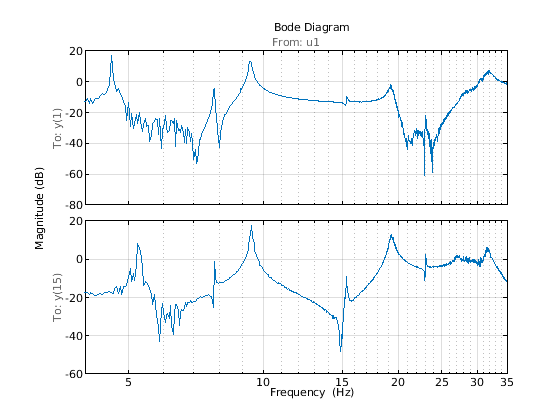The FRF shows at least 9 resonant frequencies. For analysis we want to focus on 6-35 Hz frequency span where the most critical flexible bending modes of the aircraft lie. Hence reduce the FRF to this frequency region.

```f = G.Frequency/2/pi; % extract frequency vector in Hz (G stores frequency in rad/s) Gs = fselect(G, f>6 & f<=32) % "fselect" selects the FRF in the requested range (6.5 - 35 Hz)```
```Gs = IDFRD model. Contains Frequency Response Data for 20 output(s) and 1 input(s). Response data is available at 624 frequency points, ranging from 37.96 rad/s to 201.1 rad/s. Sample time: 0.0005 seconds Output channels: 'y(1)', 'y(2)', 'y(3)', 'y(4)', 'y(5)', 'y(6)', 'y(7)', 'y(8)', 'y(9)', 'y(10)', 'y(11)', 'y(12)', 'y(13)', 'y(14)', 'y(15)', 'y(16)', 'y(17)', 'y(18)', 'y(19)', 'y(20)' Input channels: 'u1' Status: Estimated model ```

`Gs` thus contains the frequency response measurements at the 20 measurement locations. Next, identify a state-space model to fit `Gs`. The subspace estimator `n4sid` provides a quick noniterative estimate. The state-space model structure is configured as follows:

1. We estimate an 18th-order continuous-time model. The order was found after some trials with various orders and checking the resulting fit of the model to the FRF.

2. The model contains a feedthrough term (D matrix is non-zero). This is because we are limiting our analysis to $\le$ 35 Hz while the wing's bandwidth is significantly larger than that (response is significant at 35 Hz).

3. To speed up computation, we suppress computation of parameter covariance.

4. The FRF magnitude varies significantly across the frequency range. In order to ensure that the low amplitudes receive equal emphasis as the higher amplitudes, we apply a custom weighting that varies inversely as the square root of the 11th response. The choice of 11th output is somewhat arbitrary but works since all 20 FRFs have similar profiles.

We set up the estimation options for `n4sid` using `n4sidOptions`.

```FRF = squeeze(Gs.ResponseData); Weighting = mean(1./sqrt(abs(FRF))).'; n4Opt = n4sidOptions('EstimateCovariance',false,... 'WeightingFilter',Weighting,... 'OutputWeight',eye(20)); sys1 = n4sid(Gs,24,'Ts',0,'Feedthrough',true,n4Opt); Fit = sys1.Report.Fit.FitPercent'```
```Fit = 1×20 57.0200 57.9879 85.0160 86.3815 80.4879 80.4430 58.2216 45.2692 61.5057 76.7612 84.7305 86.2600 86.4266 85.0251 76.9208 82.1191 74.7982 79.6837 67.9078 76.7249 ```

The "Fit" numbers shows the percentage fit between the data (`Gs`) and model's (`sys1`) frequency response using a normalized root-mean-square-error (NRMSE) goodness-of-fit measure. The poorest and best fits are plotted next.

```[~,Imin] = min(Fit); [~,Imax] = max(Fit); clf bodeplot(Gs([Imin, Imax],:), sys1([Imin, Imax],:), opt); xlim([6 32]) title('Worst (top) and best (bottom) fits between data and model') grid on legend('Data', 'Model')```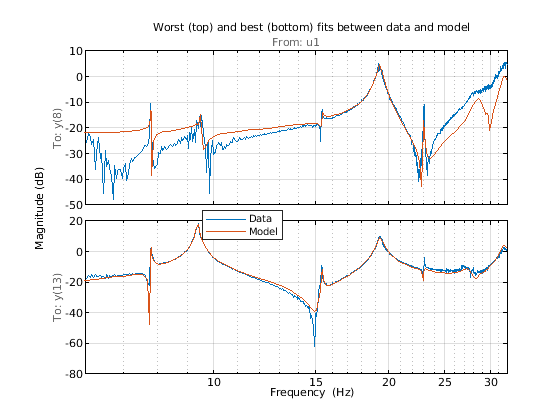The fits achieved with model `sys1` can be improved even more by iterative nonlinear least-squares refinement of model's parameters. This can be achieved using the `ssest` command. We set up the estimation options for `ssest` using the `ssestOptions` command. This time the parameter covariance is also estimated.

```ssOpt = ssestOptions('EstimateCovariance',true,... 'WeightingFilter',n4Opt.WeightingFilter,... 'SearchMethod','lm',... % use Levenberg-Marquardt search method 'Display','on',... 'OutputWeight',eye(20)); sys2 = ssest(Gs, sys1, ssOpt); % estimate state-space model (this takes several minutes) Fit = sys2.Report.Fit.FitPercent'```
```Fit = 1×20 89.7225 89.5185 89.7260 90.4986 88.5522 88.8727 81.3225 83.5975 75.9215 83.1763 91.1358 89.7310 90.6844 89.8444 89.6685 89.1467 87.8532 88.0385 84.2898 83.3578 ```

As before we plot the worst and the best fits. We also visualize the 1-sd confidence region for the model's frequency response.

```[~, Imin] = min(Fit); [~, Imax] = max(Fit); clf h = bodeplot(Gs([Imin, Imax],:), sys2([Imin, Imax],:), opt); showConfidence(h, 1) xlim([6.5 35]) title('Worst (top) and best (bottom) fits between data and refined model') grid on legend('Data', 'Model (sys2)')```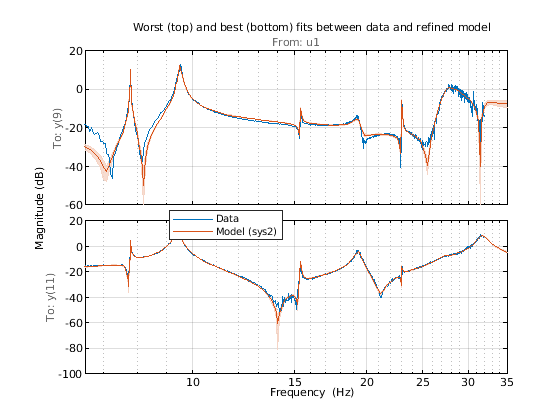The refined state-space model `sys2` fits the FRFs quite well in the 7–20 Hz region. The uncertainty bounds are tight around most resonant locations. We estimated a 24th-order model which means that there are at most 12 oscillatory modes in the system `sys2`. Use the `modalfit` command to fetch the natural frequencies in Hz for these modes.

```f = Gs.Frequency/2/pi; % data frequencies (Hz) fn = modalfit(sys2, f, 12); % natural frequencies (Hz) disp(fn)```
``` 7.7721 7.7953 9.3147 9.4009 9.4910 15.3463 19.3291 23.0219 27.4164 28.7256 31.7014 63.3034 ```

The values in `fn` suggest two frequencies very close to 7.8 Hz and three close to 9.4 Hz. An inspection of frequency responses near these frequencies reveals that the peaks location shift a little bit across outputs. These discrepancies may be removed by better control over the experiment process, performing direct time-domain identification with input bandwidth limited to narrow range centered at these frequencies, or fitting a single oscillatory mode to the frequency response around these frequencies. These alternatives are not explored in this example.

### Modal Parameter Computation

We can now use the model `sys2` to extract the modal parameters. An inspection of the FRFs indicates around 10 modal frequencies, roughly around the frequencies [5 7 10 15 17 23 27 30] Hz. We can make this assessment more concrete by using the `modalsd` command that checks the stability of modal parameters as the order of the underlying model is changed. This operation takes a long time. Hence the resulting plot is inserted directly as an image. Execute the code inside the comment block below to reproduce the figure.

```FRF = permute(Gs.ResponseData,[3 1 2]); f = Gs.Frequency/2/pi; %{ figure pf = modalsd(FRF,f,fs,'MaxModes',20,'FitMethod','lsrf'); %}```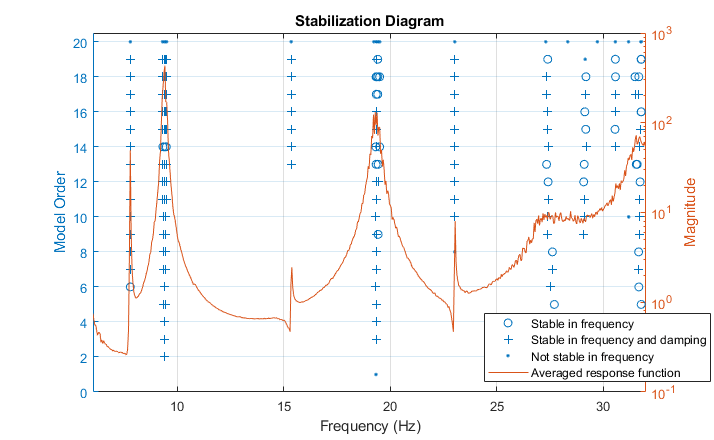Inspection of the plot and `pf` values suggests a refined list of true natural frequencies:

`Freq = [7.8 9.4 15.3 19.3 23.0 27.3 29.2 31.7];`

We use the values in `Freq` vector as a guide for picking most dominant modes from the model `sys2`. This is done using the `modalfit` command.

`[fn,dr,ms] = modalfit(sys2,f,length(Freq),'PhysFreq',Freq);`

`fn` are the natural frequencies (in Hz), `dr` the corresponding damping coefficients and `ms` the normalized residuals as column vectors (one column for each natural frequency). In the process of extraction of these modal parameters, only the stable, under-damped poles of the model are used. The `ms` columns contain data for only the poles with positive imaginary part.

### Mode Shape Visualization

To visualize the various bending modes, we need the modal parameters extracted above. In addition we need information on the location of the measurement points. These positions (x-y values) is recorded for each accelerometer in the matrix `AccePos`:

```AccelPos = [... % see figure 2 16.63 18.48; % nearest right of center 16.63 24.48; 27.90 22.22; 27.90 28.22; 37.17 25.97; 37.17 31.97; 46.44 29.71; 46.44 35.71; 55.71 33.46; 55.71 39.46; % farthest right -16.63 18.48; % nearest left of center -16.63 24.18; -27.90 22.22; -27.90 28.22; -37.17 25.97; -37.17 31.97; -46.44 29.71; -46.44 35.71; -55.71 33.46; -55.71 39.46]; % farthest left```

The mode shapes are contained in the matrix `ms` where each column corresponds to the shape for one frequency. Animate the mode by superimposing mode shape amplitudes over the sensor coordinates and varying the amplitudes at the mode's natural frequency. The animation shows the bending with no damping. As an example, consider the mode at 15.3 Hz.

`AnimateOneMode(3, fn, ms, AccelPos); `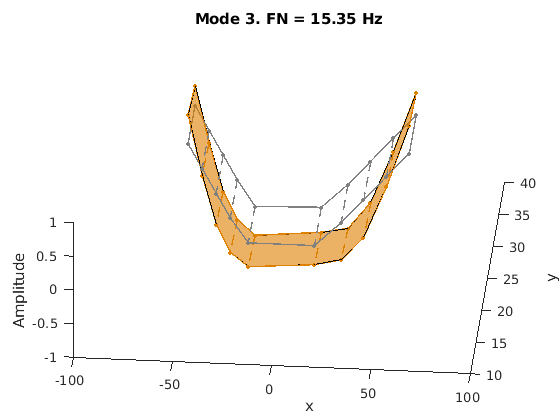### Conclusions

This example shows a parametric model identification based approach to modal parameter estimation. Using a state-space model of 24th order, 8 stable oscillatory modes in the frequency region 6–32 Hz were extracted. The modal information was combined with the knowledge of the accelerometer positions to visualize the various bending modes. Some of these modes are shown in the figures below.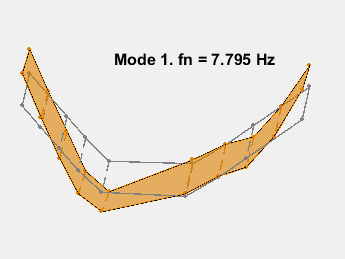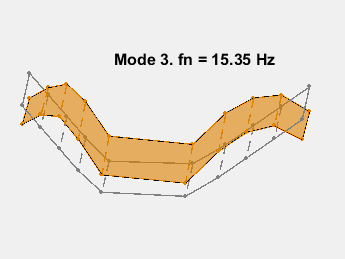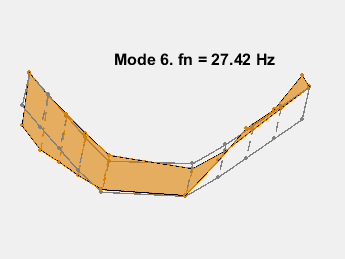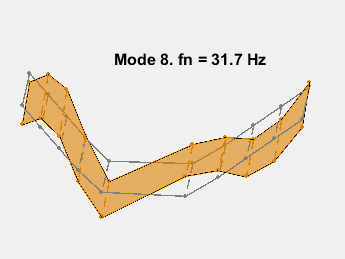### References

 Gupta, Abhineet, Peter J. Seiler, and Brian P. Danowsky. "Ground Vibration Tests on a Flexible Flying Wing Aircraft." AIAA Atmospheric Flight Mechanics Conference, AIAA SciTech Forum. (AIAA 2016-1753).

```function AnimateOneMode(ModeNum, fn, ModeShapes, AccelPos) % Animate one mode. % ModeNum: Index of the mode. % Reorder the sensor locations for plotting so that a continuous, % non-intersecting curve is traced around the body of the aircraft. PlotOrder = [19:-2:11, 1:2:9, 10:-2:2, 12:2:20, 19]; Fwd = PlotOrder(1:10); Aft = PlotOrder(20:-1:11); x = AccelPos(PlotOrder,1); y = AccelPos(PlotOrder,2); xAft = AccelPos(Aft,1); yAft = AccelPos(Aft,2); xFwd = AccelPos(Fwd,1); yFwd = AccelPos(Fwd,2); wn = fn(ModeNum)*2*pi; % Mode frequency in rad/sec T = 1/fn(ModeNum); % Period of modal oscillation Np = 2.25; % Number of periods to simulate tmax = Np*T; % Simulate Np periods Nt = 100; % Number of time steps for animation t = linspace(0,tmax,Nt); ThisMode = ModeShapes(:,ModeNum)/max(abs(ModeShapes(:,ModeNum))); % normalized for plotting z0 = ThisMode(PlotOrder); zFwd = ThisMode(Fwd); zAft = ThisMode(Aft); clf col1 = [1 1 1]*0.5; xx = reshape([[xAft, xFwd]'; NaN(2,10)],[2 20]); yy = reshape([[yAft, yFwd]'; NaN(2,10)],[2 20]); plot3(x,y,0*z0,'-', x,y,0*z0,'.', xx(:), yy(:), zeros(40,1),'--',... 'Color',col1,'LineWidth',1,'MarkerSize',10,'MarkerEdgeColor',col1); xlabel('x') ylabel('y') zlabel('Amplitude') ht = max(abs(z0)); axis([-100 100 10 40 -ht ht]) view(5,55) title(sprintf('Mode %d. FN = %s Hz', ModeNum, num2str(fn(ModeNum),4))); % Animate by updating z-coordinates. hold on col2 = [0.87 0.5 0]; h1 = plot3(x,y,0*z0,'-', x,y,0*z0,'.', xx(:), yy(:), zeros(40,1),'--',... 'Color',col2,'LineWidth',1,'MarkerSize',10,'MarkerEdgeColor',col2); h2 = fill3(x,y,0*z0,col2,'FaceAlpha',0.6); hold off for k = 1:Nt Rot1 = cos(wn*t(k)); Rot2 = sin(wn*t(k)); z_t = real(z0)*Rot1 - imag(z0)*Rot2; zAft_t = real(zAft)*Rot1 - imag(zAft)*Rot2; zFwd_t = real(zFwd)*Rot1 - imag(zFwd)*Rot2; zz = reshape([[zAft_t, zFwd_t]'; NaN(2,10)],[2 20]); set(h1(1),'ZData',z_t) set(h1(2),'ZData',z_t) set(h1(3),'ZData',zz(:)) h2.Vertices(:,3) = z_t; pause(0.1) end end ```
##### Support평가판 신청